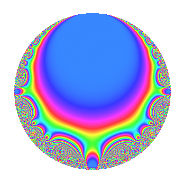# Properties

 Label 14.5.dLevel 14 Weight 5 Character orbit d Rep. character $$\chi_{14}(3,\cdot)$$ Character field $$\Q(\zeta_{6})$$ Dimension 4 Newforms 1 Sturm bound 10 Trace bound 0

# Related objects

## Defining parameters

 Level: $$N$$ = $$14 = 2 \cdot 7$$ Weight: $$k$$ = $$5$$ Character orbit: $$[\chi]$$ = 14.d (of order $$6$$ and degree $$2$$) Character conductor: $$\operatorname{cond}(\chi)$$ = $$7$$ Character field: $$\Q(\zeta_{6})$$ Newforms: $$1$$ Sturm bound: $$10$$ Trace bound: $$0$$

## Dimensions

The following table gives the dimensions of various subspaces of $$M_{5}(14, [\chi])$$.

Total New Old
Modular forms 20 4 16
Cusp forms 12 4 8
Eisenstein series 8 0 8

## Trace form

 $$4q$$ $$\mathstrut -\mathstrut 18q^{3}$$ $$\mathstrut -\mathstrut 16q^{4}$$ $$\mathstrut +\mathstrut 54q^{5}$$ $$\mathstrut -\mathstrut 28q^{7}$$ $$\mathstrut +\mathstrut 84q^{9}$$ $$\mathstrut +\mathstrut O(q^{10})$$ $$4q$$ $$\mathstrut -\mathstrut 18q^{3}$$ $$\mathstrut -\mathstrut 16q^{4}$$ $$\mathstrut +\mathstrut 54q^{5}$$ $$\mathstrut -\mathstrut 28q^{7}$$ $$\mathstrut +\mathstrut 84q^{9}$$ $$\mathstrut -\mathstrut 96q^{10}$$ $$\mathstrut -\mathstrut 54q^{11}$$ $$\mathstrut +\mathstrut 144q^{12}$$ $$\mathstrut -\mathstrut 708q^{15}$$ $$\mathstrut -\mathstrut 128q^{16}$$ $$\mathstrut +\mathstrut 918q^{17}$$ $$\mathstrut +\mathstrut 576q^{18}$$ $$\mathstrut +\mathstrut 30q^{19}$$ $$\mathstrut -\mathstrut 378q^{21}$$ $$\mathstrut +\mathstrut 192q^{22}$$ $$\mathstrut -\mathstrut 486q^{23}$$ $$\mathstrut -\mathstrut 768q^{24}$$ $$\mathstrut -\mathstrut 572q^{25}$$ $$\mathstrut -\mathstrut 1728q^{26}$$ $$\mathstrut +\mathstrut 1456q^{28}$$ $$\mathstrut +\mathstrut 3240q^{29}$$ $$\mathstrut +\mathstrut 1152q^{30}$$ $$\mathstrut -\mathstrut 546q^{31}$$ $$\mathstrut +\mathstrut 1062q^{33}$$ $$\mathstrut -\mathstrut 1890q^{35}$$ $$\mathstrut -\mathstrut 1344q^{36}$$ $$\mathstrut -\mathstrut 446q^{37}$$ $$\mathstrut -\mathstrut 4320q^{38}$$ $$\mathstrut -\mathstrut 3312q^{39}$$ $$\mathstrut +\mathstrut 768q^{40}$$ $$\mathstrut +\mathstrut 5376q^{42}$$ $$\mathstrut +\mathstrut 2344q^{43}$$ $$\mathstrut -\mathstrut 432q^{44}$$ $$\mathstrut +\mathstrut 5724q^{45}$$ $$\mathstrut +\mathstrut 3840q^{46}$$ $$\mathstrut +\mathstrut 702q^{47}$$ $$\mathstrut -\mathstrut 9212q^{49}$$ $$\mathstrut -\mathstrut 3456q^{50}$$ $$\mathstrut +\mathstrut 318q^{51}$$ $$\mathstrut -\mathstrut 384q^{52}$$ $$\mathstrut +\mathstrut 2754q^{53}$$ $$\mathstrut -\mathstrut 1440q^{54}$$ $$\mathstrut -\mathstrut 17460q^{57}$$ $$\mathstrut +\mathstrut 384q^{58}$$ $$\mathstrut +\mathstrut 12366q^{59}$$ $$\mathstrut +\mathstrut 2832q^{60}$$ $$\mathstrut +\mathstrut 7686q^{61}$$ $$\mathstrut +\mathstrut 6468q^{63}$$ $$\mathstrut +\mathstrut 2048q^{64}$$ $$\mathstrut -\mathstrut 3024q^{65}$$ $$\mathstrut -\mathstrut 3456q^{66}$$ $$\mathstrut -\mathstrut 5062q^{67}$$ $$\mathstrut -\mathstrut 7344q^{68}$$ $$\mathstrut -\mathstrut 2016q^{70}$$ $$\mathstrut +\mathstrut 18792q^{71}$$ $$\mathstrut +\mathstrut 4608q^{72}$$ $$\mathstrut -\mathstrut 17274q^{73}$$ $$\mathstrut -\mathstrut 4320q^{74}$$ $$\mathstrut -\mathstrut 5220q^{75}$$ $$\mathstrut +\mathstrut 4914q^{77}$$ $$\mathstrut +\mathstrut 8832q^{78}$$ $$\mathstrut +\mathstrut 794q^{79}$$ $$\mathstrut -\mathstrut 3456q^{80}$$ $$\mathstrut -\mathstrut 4338q^{81}$$ $$\mathstrut +\mathstrut 9984q^{82}$$ $$\mathstrut -\mathstrut 5040q^{84}$$ $$\mathstrut +\mathstrut 10380q^{85}$$ $$\mathstrut -\mathstrut 10368q^{86}$$ $$\mathstrut -\mathstrut 12276q^{87}$$ $$\mathstrut -\mathstrut 768q^{88}$$ $$\mathstrut -\mathstrut 12474q^{89}$$ $$\mathstrut +\mathstrut 2688q^{91}$$ $$\mathstrut +\mathstrut 7776q^{92}$$ $$\mathstrut +\mathstrut 18918q^{93}$$ $$\mathstrut +\mathstrut 15168q^{94}$$ $$\mathstrut +\mathstrut 8910q^{95}$$ $$\mathstrut +\mathstrut 6144q^{96}$$ $$\mathstrut -\mathstrut 11448q^{99}$$ $$\mathstrut +\mathstrut O(q^{100})$$

## Decomposition of $$S_{5}^{\mathrm{new}}(14, [\chi])$$ into irreducible Hecke orbits

Label Dim. $$A$$ Field CM Traces $q$-expansion
$$a_2$$ $$a_3$$ $$a_5$$ $$a_7$$
14.5.d.a $$4$$ $$1.447$$ $$\Q(\sqrt{2}, \sqrt{-3})$$ None $$0$$ $$-18$$ $$54$$ $$-28$$ $$q+\beta _{1}q^{2}+(-6+2\beta _{1}-3\beta _{2}-2\beta _{3})q^{3}+\cdots$$

## Decomposition of $$S_{5}^{\mathrm{old}}(14, [\chi])$$ into lower level spaces

$$S_{5}^{\mathrm{old}}(14, [\chi]) \cong$$ $$S_{5}^{\mathrm{new}}(7, [\chi])$$$$^{\oplus 2}$$